# Estimate tail dependence coefficients on the wave-surge data¶

In this example we estimate the tail dependence coefficient of a bivariate sample applied to the concurrent measurements of two oceanographic variables (wave and surge heights) at a single location off south-west England. Readers should refer to [coles2001] to get more details.

First, we load the wave-surge dataset.

import openturns as ot
import openturns.viewer as otv
from openturns.usecases import coles

data = coles.Coles().wavesurge
print(data[:5])

graph = ot.Graph(
"Concurent wave and surge heights", "wave (m)", "surge (m)", True, ""
)
cloud = ot.Cloud(data)
cloud.setColor("red")
view = otv.View(graph)[ wave   surge  ]
0 : [  1.5   -0.009 ]
1 : [  1.83  -0.053 ]
2 : [  2.44  -0.024 ]
3 : [  1.68   0     ]
4 : [  1.49   0.079 ]


We plot the graph of the function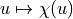and the graph of the function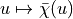. We conclude that both variables are asymptotially dependent as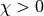and that they are positively correlated as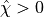. We can visually deduce the upper tail dependence coefficient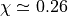and the upper extremal dependence coefficient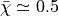.

graph1 = ot.VisualTest.DrawUpperTailDependenceFunction(data)
graph2 = ot.VisualTest.DrawUpperExtremalDependenceFunction(data)
grid = ot.GridLayout(1, 2)
grid.setGraph(0, 0, graph1)
grid.setGraph(0, 1, graph2)
view = otv.View(grid)otv.View.ShowAll()